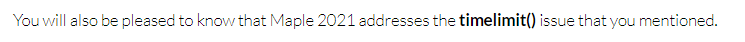# Question:timelimit still does not work for some cases in Maple 2021

## Question:timelimit still does not work for some cases in Maple 2021

Maple 2021

timelimit() has improved in Maple 2021, and I thought it works now for everything.

https://www.mapleprimes.com/maplesoftblog/213986-Introducing-Maple-Learn-officially#comment204444But unfortunately,, it still hangs on some dsolves when I was testing something. Here is an example

```restart;
ode:=(y(x)^4-a^2*x^2)*diff(y(x),x)^2+2*a^2*x*y(x)*diff(y(x),x)+y(x)^2*(y(x)^2-a^2)=0;
timelimit(60,dsolve(ode,y(x)))
```

The above has one minute timeout. But its been running for 20 minutes now. Here is another example that hangs

```restart;
ode:=(diff(y(x),x) = (-y(x)^2+4*a*x)^3/(-y(x)^2+4*a*x-1)/y(x));
timelimit(60,dsolve(ode,y(x)))```

I have found many more dsolve examples that hang with timelimit. Will update later. May be they will help find the cause.

Hopefully this timelimit issue will be fixed in some future release.  hangs in Maple commands even when using timelimit makes it very hard to run long scripts that run over many problems, since do not know when/where it will hang and have to restart and clean things manually each time.

Window 10. Maple 2021

Update March 15,2022

Here is another example of timelimit taking so much longer than asking for, it is pretty much useless.

I will use this question to collect such examples in one place. Here I asked for one minute (60 seconds, CPU time) timeout. Maple actually timedout after using 1381 CPU seconds which is 23 times as much as requested.

 > restart;
 > ode:=x^2*(x^2+1)*diff(y(x),x\$2)+7*x*exp(x)*diff(y(x),x)+9*(1+tan(x))*y(x)=0; current_time:=time(); try    timelimit(60,dsolve(ode)) catch:    print("Good. Timelimit worked"); end try; time_used := time()-current_time;> 1381/60.>

Help says

The timelimit function evaluates the expression x, but gives up if the evaluation takes longer than the number of seconds specified by t.
Note: In some cases, the execution may not abort at exactly the time limit imposed, but will abort as soon as it can do so safely.  This can happen when execution is in critical sections of certain built-in routines.

For me, the above makes no sense at all. What does "as soon as it can do safely"? I am not running the code to control an airplane flight full of people here. This is some code running on my PC at home to do some calculation. It can abort right away. It will not hurt me physically or hurt my PC to do so.

So what does "do so safely" actually mean? Safety of what exactly?

Compare the above example to Mathematica where it times out at almost exactly to the requested timeout. Here I ask for 3 seconds timeoutI am not asking for exact timeout. But asking for 1 minute timeout and getting 8 minutes is not good at all.

1.5 minutes is OK. even 2 minutes is OK.

I have more examples to add. But will do that later.

Update

Quick try with Maple 2022 shows timelimit has much improved. Here are 3 examples from above. First one asks for 60 seconds limit, it finishes in 288 seconds.   Second one asks for 60 seconds, it finishes in 96 seconds. Third one asks for 60 seconds and finishes in 73 second.

This is much much better than in Maple 2021. Thanks for the Maple engineers who improved this.

 > interface(version)> restart;
 > ode:=x^2*(x^2+1)*diff(y(x),x\$2)+7*x*exp(x)*diff(y(x),x)+9*(1+tan(x))*y(x)=0; current_time:=time(); try    timelimit(60,dsolve(ode)) catch:    print("Good. Timelimit worked"); end try; time_used := time()-current_time;> ode:=(y(x)^4-a^2*x^2)*diff(y(x),x)^2+2*a^2*x*y(x)*diff(y(x),x)+y(x)^2*(y(x)^2-a^2)=0; current_time:=time(); try    timelimit(60,dsolve(ode)) catch:    print("Good. Timelimit worked"); end try; time_used := time()-current_time;> ode:=(diff(y(x),x) = (-y(x)^2+4*a*x)^3/(-y(x)^2+4*a*x-1)/y(x)); current_time:=time(): try    timelimit(60,dsolve(ode)) catch:    print("Good. Timelimit worked"); end try; time_used := time()-current_time;>